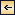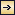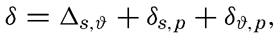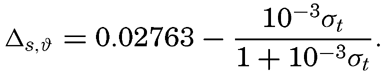Tables for Computing Geopotential Distances between Isobaric Surfaces### Symbols and Definitions

α35,0,p: Specific volume of sea water of salinity 35 ‰ and temperature O°C, and at pressure p.

δ: Anomaly of specific volume of sea water of salinity S and temperature ϑ and at pressure p (see p. 58):[Equation]
where[Equation]

(DlD2)s: Standard geopotential distance between the isobaric surfaces p1 and p2 (equation XII, 6, p. 408):

1052[Equation]

ΔD: Anomaly of the geopotential distance between the isobaric surfaces p1 and p2 (equation XII, 7, p. 409):[Equation]Tables for Computing Geopotential Distances between Isobaric Surfaces